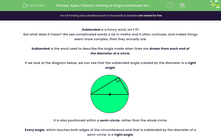# Apply Theorem relating to Angles Subtended from the Diameter

In this worksheet, students will find the value of unknown angles and solve problems requiring the application of the circle theory that angles subtended from the diameter will create a right angle.Key stage:  KS 4

Year:  GCSE

GCSE Subjects:   Maths

GCSE Boards:   OCR, AQA, Eduqas, Pearson Edexcel,

Curriculum topic:   Basic Geometry, Geometry and Measures

Curriculum subtopic:   Circles Properties and Constructions

Popular topics:   Geometry worksheets

Difficulty level:#### Worksheet Overview

Subtended is a funny word, isn't it?

But what does it mean? We see complicated words a lot in maths and it often confuses, and makes things seem more complex, than they actually are.

Subtended is the word used to describe the angle made when lines are drawn from each end of the diameter of a circle.

If we look at the diagram below, we can see that the subtended angle created by the diameter is a right angle:It is also positioned within a semi-circle, rather than the whole circle.

Every angle, which touches both edges of the circumference and that is subtended by the diameter of a semi-circle, is a right angle.

This sounds complex, but all it means is that if you draw a straight line from each end of the diameter to hit the circumference of the circle, the point where they touch will create a right angle.

Example

Find the value of angle x in the diagram below:We know that the total angles in a triangle add up to 180°.

We also know that the one angle of this triangle is a right angle (90°).

We have been given one other angle (38°).

If we put these facts together, we can subtract the two known angles from 180° to find x:

180 - 90 - 38 = 52°

A quick tip before we start, circle theorem questions rarely show the right angle depicted by the square in the corner. Instead, it will be assumed that you know that angles subtended from the diameter will create 90°.

In this activity, we will find the value of unknown angles and solve problems requiring the application of the theory explained above.

### What is EdPlace?

We're your National Curriculum aligned online education content provider helping each child succeed in English, maths and science from year 1 to GCSE. With an EdPlace account you’ll be able to track and measure progress, helping each child achieve their best. We build confidence and attainment by personalising each child’s learning at a level that suits them.

Get started